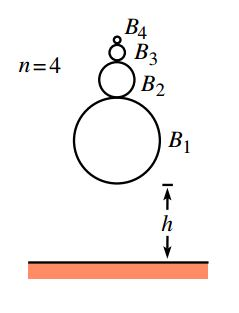Now consider $n$ balls, $B_1, . . . , B_n$, having masses $m_1, m_2, . . . , m_n$ $(with~ m_1 >>m_2 >>· · ·>>m_n)$, sitting in a vertical stack. The bottom of $B_1$ is a height $h$ above the ground, and the bottom of $B_n$ is a height $h + l$ above the ground. The balls are dropped. In terms of $n$, to what height does the top ball bounce?(Note: Work in the approximation where $m_1$ is much larger than $m_2$, which is much larger than $m_3$, etc., and assume that the balls bounce elastically.)

Try part 1 also - Basketball and tennis ball!

Question Source - Basketball and tennis ball

×